# Machine Learning Part 7 — Forward propagation in neural net in SQL

This is the seventh part of the ML series. For your convenience you can find other parts in the table of contents in Part 1 – Linear regression in MXNet

Today we are going to create a neural net and calculate forward propagation using PostgreSQL. Let’s go.

We start with definition of the network: we will have input layer, hidden layer, and output layer. Input layer will have 3 nodes, hidden layer will have 2, output layer will have 3. In input layer we don’t do any transformation on the input data, in hidden layer we use ReLU, in output layer we use linear activation function (so no transformation).

We define some input values, weights and biases. Values are completely made up and do not make a difference.

Before we write SQL code, let’s calculate result manually.

We have the following variables: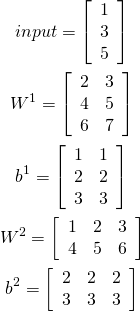Now, let’s calculate input for hidden layer: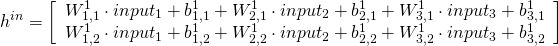Now, we use ReLU activation function for hidden layer: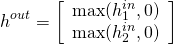We carry on with calculating input for output layer: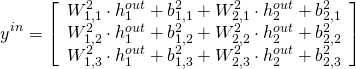Activation function for output layer is linear, so it is easy now: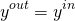We will calculate errors next time.

Now, let’s calculate the result:

This is actually very easy. We divide the process into multiple phases. Each row of CTE represents one complete path from some input node to some output node. Initially row carries some metadata and input value, in each phase we fill some next value using different case expressions.

In phase 0 we get the input and transform it into output, since input layer has no logic, we just copy the value.
In phase 1 we calculate inputs for next layer by multiplying weights and values.
In phase 2 we activate hidden layer. Since we use ReLU, we perform a very simple comparison.
In phase 3 we once again use weights and values to calculate input for next layer, this time we use different weights.
In phase 4 we activate output layer, which just copies values (since we use a linear activation function).

So in our query we start by defining a schema. We simply join all tables and cross join dummy table with one row which we use to define additional column. We fill these columns later throughout the process.

In recursive part of CTE we simply either rewrite values or do some logic depending on the phase number.

Next time we will see how to backpropagate errors.